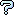All about flooble | fun stuff | Get a free chatterbox | Free JavaScript | Avatarsperplexus dot infoEqual Angles (Posted on 2005-12-14)Let circle A be in the interior of circle B and tangent to it at point M. Let chord QR of circle B be tangent to circle A at point P. Prove that angles PMQ and PMR are equal.

1993 British Mathematical Olympiad,Round 1,Problem 4.

 Submitted by Bractals No Rating Solution: (Hide) ```Let circles A and B have radii a and b respectively. Letting M be the origin and using polar coordinates the circles have the following equations: Circle A: r = 2a cos(theta) Circle B: r = 2b cos(theta) where theta is the angle between r and the line determined by the centers of the circles. Let Q' be the intersection of MQ and circle A. Let R' be the intersection of MR and circle A. MQ' 2a cos(theta_q) a 2a cos(theta_r) MR' ----- = ----------------- = --- = ----------------- = ----- MQ 2b cos(theta_q) b 2b cos(theta_r) MR ==> triangles Q'MR' and QMR are similar ==> Q'R' and QR are parallel ==> AP and Q'R' are perpendicular ==>Comments: ( You must be logged in to post comments.)
 Subject Author DateTo elaborate DrBob 2005-12-15 10:44:19re: re is there an easier way DrBob 2005-12-15 10:42:25re: Is there an easier way? ??? Jer 2005-12-14 14:28:39Is there an easier way? DrBob 2005-12-14 12:15:09Please log in:
 Login: Password: Remember me: Sign up! | Forgot password

 Search: Search body:
Forums (0)
Newest Problems
Random Problem
FAQ | About This Site
Site Statistics
New Comments (1)
Unsolved Problems
Top Rated Problems
This month's top
Most Commented On

Chatterbox:
Copyright © 2002 - 2019 by Animus Pactum Consulting. All rights reserved. Privacy Information